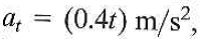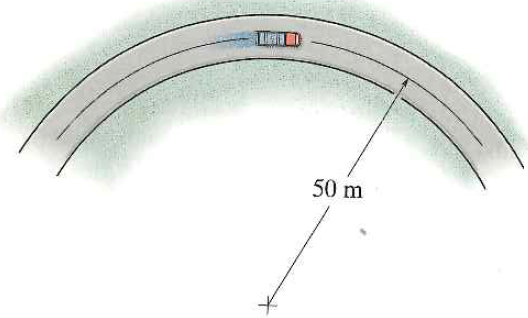Problem

# The truck travels along a circular road that has a radius of 50 m at a speed of 4 m/s. F...

The truck travels along a circular road that has a radius of 50 m at a speed of 4 m/s. For a short distance when t = 0, its speed is then increased bywhere t is in seconds. Determine the speed and the magnitude of the truck's acceleration when t = 4 s.#### Step-by-Step Solution

Solution 1

Given

Radius of the path, $$\rho=50 \mathrm{~m}$$

Initial speed, $$v_{1}=4 \mathrm{~m} / \mathrm{s}$$

Acceleration, $$a_{t}=(0.4 t) \mathrm{m} / \mathrm{s}^{2}$$

\begin{aligned} &a_{t}=0.4 t \\ &\frac{d v}{d t}=0.4 t \Rightarrow d v=0.4 t d t \\ &\int_{4}^{v} d v=0.4 \int_{0}^{t} t d t \\ &v-4=0.2 t^{2} \\ &v=4+0.2 t^{2} \end{aligned}

when $$t=4 \mathrm{~s}$$

$$v=4+0.2(4)^{2}=7.2 \mathrm{~m} / \mathrm{s}$$

Normal aceleration, $$a_{n}=\frac{v^{2}}{\rho}=\frac{7.2^{2}}{50}=1.0368 \mathrm{~m} / \mathrm{s}^{2}$$

Tangential aceleration, $$a_{4}=(0.4 \times 4)=1.6 \mathrm{~m} / \mathrm{s}^{2}$$

Aceleration, $$a=\sqrt{a_{n}{ }^{2}+a_{t}{ }^{2}}=\sqrt{1.0368^{2}+1.6^{2}}=1.9065 \mathrm{~m} / \mathrm{s}^{2}$$